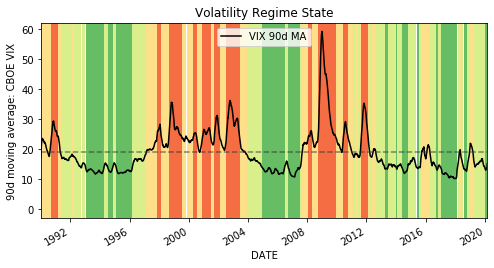# Real Python Matplotlib Tutorial

### Resources¶

In :
import matplotlib.pyplot as plt

In :
import numpy as np

In :
np.random.seed(444)

• One important big-picture matplotlib concept is its object hierarchy.

• A “hierarchy” here means that there is a tree-like structure of matplotlib objects underlying each plot.

• A Figure object is the outermost container for a matplotlib graphic, which can contain multiple Axes objects. One source of confusion is the name: an Axes actually translates into what we think of as an individual plot or graph (rather than the plural of “axis,” as we might expect).

In :
fig, _ = plt.subplots()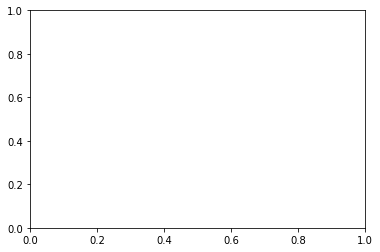In :
type(one_tick := fig.axes.yaxis.get_major_ticks())

Out:
matplotlib.axis.YTick
• Notice that we didn’t pass arguments to subplots() here. The default call is subplots(nrows=1, ncols=1)

In :
fig, ax = plt.subplots()In :
type(ax)

Out:
matplotlib.axes._subplots.AxesSubplot
• We can call its instance methods to manipulate the plot similarly to how we call pyplots functions. Let’s illustrate with a stacked area graph of three time series

In :
(rng := np.arange(50))

Out:
array([ 0,  1,  2,  3,  4,  5,  6,  7,  8,  9, 10, 11, 12, 13, 14, 15, 16,
17, 18, 19, 20, 21, 22, 23, 24, 25, 26, 27, 28, 29, 30, 31, 32, 33,
34, 35, 36, 37, 38, 39, 40, 41, 42, 43, 44, 45, 46, 47, 48, 49])
In :
(rnd := np.random.randint(0, 10, size=(3, rng.size)))

Out:
array([[3, 0, 7, 8, 3, 4, 7, 6, 8, 9, 2, 2, 2, 0, 3, 8, 0, 6, 6, 0, 3, 0,
6, 7, 9, 3, 8, 7, 3, 2, 6, 9, 2, 9, 8, 9, 3, 2, 2, 8, 1, 5, 6, 7,
6, 0, 0, 0, 0, 4],
[8, 1, 9, 8, 5, 8, 9, 4, 6, 6, 4, 1, 8, 2, 7, 9, 3, 4, 2, 5, 0, 0,
8, 1, 0, 9, 9, 3, 2, 7, 6, 0, 5, 5, 4, 8, 3, 4, 9, 4, 7, 1, 5, 4,
4, 0, 2, 2, 5, 8],
[5, 6, 6, 1, 1, 6, 8, 4, 1, 0, 9, 2, 3, 7, 3, 3, 2, 7, 8, 6, 6, 7,
5, 7, 3, 9, 1, 3, 0, 4, 7, 5, 1, 5, 1, 4, 9, 7, 2, 4, 3, 7, 9, 2,
2, 0, 1, 5, 2, 4]])
In :
(yrs := 1950 + rng)

Out:
array([1950, 1951, 1952, 1953, 1954, 1955, 1956, 1957, 1958, 1959, 1960,
1961, 1962, 1963, 1964, 1965, 1966, 1967, 1968, 1969, 1970, 1971,
1972, 1973, 1974, 1975, 1976, 1977, 1978, 1979, 1980, 1981, 1982,
1983, 1984, 1985, 1986, 1987, 1988, 1989, 1990, 1991, 1992, 1993,
1994, 1995, 1996, 1997, 1998, 1999])
In :
fig, ax = plt.subplots(figsize=(5, 3))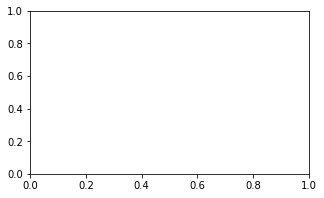In :
ax.stackplot(yrs, rng + rnd, labels=["Eastasia", "Eurasia", "Oceania"])

Out:
[<matplotlib.collections.PolyCollection at 0x7ff3b986e640>,
<matplotlib.collections.PolyCollection at 0x7ff3b9842c30>,
<matplotlib.collections.PolyCollection at 0x7ff3b981aaf0>]
In :
ax.set_title("Combined debt growth over time")

Out:
Text(0.5, 1, 'Combined debt growth over time')
In :
ax.legend(loc="upper left")

Out:
<matplotlib.legend.Legend at 0x7ff3b97ccfa0>
In :
ax.set_ylabel("Total debt")

Out:
Text(3.200000000000003, 0.5, 'Total debt')
In :
ax.set_xlim(xmin=yrs, xmax=yrs[-1])

Out:
(1950, 1999)
In :
fig.tight_layout()

In :
fig

Out: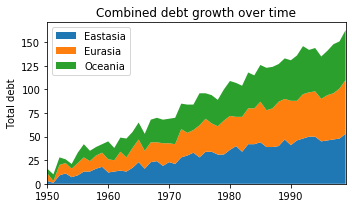Let’s look at an example with multiple subplots (Axes) within one Figure, plotting two correlated arrays that are drawn from the discrete uniform distribution:

In :
(x := np.random.randint(low=1, high=11, size=50))

Out:
array([ 9,  1,  5,  6, 10,  9,  7,  7, 10,  6,  8,  6,  4,  9,  3,  7,  6,
9,  2, 10,  7,  2,  2,  5,  7,  9,  5,  9,  9,  8,  6,  3,  4,  3,
1,  1,  5,  7,  6,  4,  4,  1,  9,  5, 10,  3,  5,  4,  1,  9])
In :
(y := x + np.random.randint(1, 5, size=x.size))

Out:
array([11,  5,  6,  7, 14, 13,  8, 10, 11,  8, 10,  7,  6, 11,  6, 10,  8,
13,  6, 11, 10,  6,  6,  9,  9, 13,  8, 12, 12, 11,  9,  4,  6,  5,
4,  2,  9,  8,  7,  8,  6,  3, 13,  8, 12,  4,  9,  7,  4, 11])
In :
(data := np.column_stack((x, y)))

Out:
array([[ 9, 11],
[ 1,  5],
[ 5,  6],
[ 6,  7],
[10, 14],
[ 9, 13],
[ 7,  8],
[ 7, 10],
[10, 11],
[ 6,  8],
[ 8, 10],
[ 6,  7],
[ 4,  6],
[ 9, 11],
[ 3,  6],
[ 7, 10],
[ 6,  8],
[ 9, 13],
[ 2,  6],
[10, 11],
[ 7, 10],
[ 2,  6],
[ 2,  6],
[ 5,  9],
[ 7,  9],
[ 9, 13],
[ 5,  8],
[ 9, 12],
[ 9, 12],
[ 8, 11],
[ 6,  9],
[ 3,  4],
[ 4,  6],
[ 3,  5],
[ 1,  4],
[ 1,  2],
[ 5,  9],
[ 7,  8],
[ 6,  7],
[ 4,  8],
[ 4,  6],
[ 1,  3],
[ 9, 13],
[ 5,  8],
[10, 12],
[ 3,  4],
[ 5,  9],
[ 4,  7],
[ 1,  4],
[ 9, 11]])
In :
fig, (ax1, ax2) = plt.subplots(nrows=1, ncols=2, figsize=(8, 4))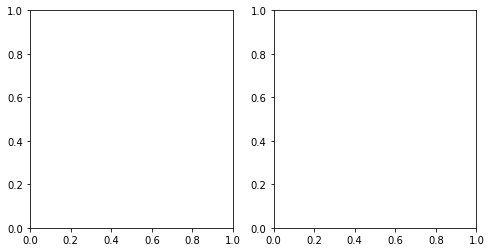In :
ax1.scatter(x=x, y=y, marker="o", c="r", edgecolor="b")

Out:
<matplotlib.collections.PathCollection at 0x7ff3bb7f7a00>
In :
ax1.set_title("Scatter: $x$ versus $y$")

Out:
Text(0.5, 1, 'Scatter: $x$ versus $y$')
In :
ax1.set_xlabel("$x$")

Out:
Text(0.5, 3.1999999999999993, '$x$')
In :
ax1.set_ylabel("$y$")

Out:
Text(3.200000000000003, 0.5, '$y$')
In :
ax2.hist(data, bins=np.arange(data.min(), data.max()), label=("x", "y"))

Out:
([array([5., 3., 4., 5., 6., 6., 6., 2., 9., 4., 0., 0.]),
array([0., 1., 1., 4., 2., 8., 4., 7., 5., 4., 6., 7.])],
array([ 1,  2,  3,  4,  5,  6,  7,  8,  9, 10, 11, 12, 13]),
<a list of 2 Lists of Patches objects>)
In :
ax2.legend(loc=(0.65, 0.8))

Out:
<matplotlib.legend.Legend at 0x7ff3bb794dc0>
In :
ax2.set_title("Frequencies of $x$ and $y$")

Out:
Text(0.5, 1, 'Frequencies of $x$ and $y$')
In :
ax2.yaxis.tick_right()

• Text inside dollar signs utilizes TeXmarkup to put variables in italics.

• Because we’re creating a “1x2” Figure, the returned result of plt.subplots(1, 2) is now a Figure object and a NumPy array of Axes objects. (You can inspect this with fig, axs = plt.subplots(1, 2) and taking a look at axs.)

In :
fig

Out: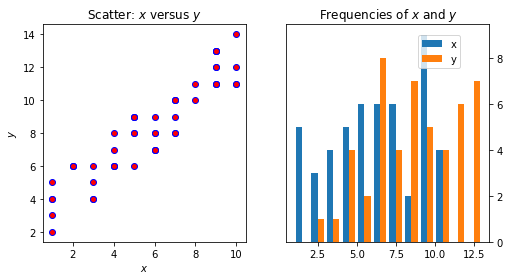In :
tuple(fig.axes[i] is ax for i, ax in zip(range(2), (ax1, ax2)))

Out:
(True, True)
• Taking this one step further, we could alternatively create a figure that holds a 2x2 grid of Axes objects

In :
fig, ax = plt.subplots(nrows=2, ncols=2, figsize=(7, 7))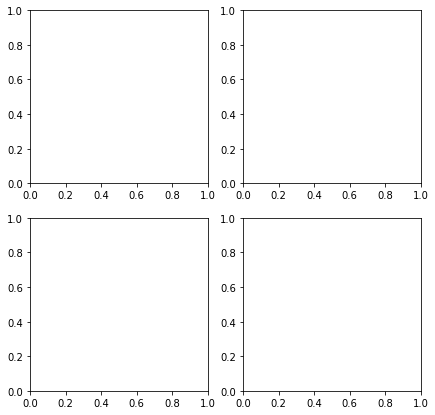• Now, what is ax? It’s no longer a single Axes, but a two-dimensional NumPy array of them

In :
type(ax)

Out:
numpy.ndarray
In :
ax

Out:
array([[<matplotlib.axes._subplots.AxesSubplot object at 0x7ff3b97b0a00>,
<matplotlib.axes._subplots.AxesSubplot object at 0x7ff3b975d3c0>],
[<matplotlib.axes._subplots.AxesSubplot object at 0x7ff3b971a730>,
<matplotlib.axes._subplots.AxesSubplot object at 0x7ff3b97da410>]],
dtype=object)
In :
ax1, ax2, ax3, ax4 = ax.flatten()


To illustrate some more advanced subplot features, let’s pull some macroeconomic California housing data extracted from a compressed tar archive, using io, tarfile, and urllib from Python’s Standard Library.

In :
import tarfile
from urllib.request import urlretrieve
from pathlib import Path

In :
filepath, response = urlretrieve(
"http://www.dcc.fc.up.pt/~ltorgo/Regression/cal_housing.tgz"
)

In :
filepath

Out:
'/tmp/tmpyh987137'
In :
with tarfile.open(name=filepath, mode="r") as archive:
archive.extractfile("CaliforniaHousing/cal_housing.data"), delimiter=","
)

In :
housing

Out:
array([[-1.2223e+02,  3.7880e+01,  4.1000e+01, ...,  1.2600e+02,
8.3252e+00,  4.5260e+05],
[-1.2222e+02,  3.7860e+01,  2.1000e+01, ...,  1.1380e+03,
8.3014e+00,  3.5850e+05],
[-1.2224e+02,  3.7850e+01,  5.2000e+01, ...,  1.7700e+02,
7.2574e+00,  3.5210e+05],
...,
[-1.2122e+02,  3.9430e+01,  1.7000e+01, ...,  4.3300e+02,
1.7000e+00,  9.2300e+04],
[-1.2132e+02,  3.9430e+01,  1.8000e+01, ...,  3.4900e+02,
1.8672e+00,  8.4700e+04],
[-1.2124e+02,  3.9370e+01,  1.6000e+01, ...,  5.3000e+02,
2.3886e+00,  8.9400e+04]])
In :
(y := housing[:, -1])

Out:
array([452600., 358500., 352100., ...,  92300.,  84700.,  89400.])

The property T is an accessor to the method transpose().

In :
pop, age = housing[:, [4, 7]].T

In :
pop, age

Out:
(array([ 129., 1106.,  190., ...,  485.,  409.,  616.]),
array([8.3252, 8.3014, 7.2574, ..., 1.7   , 1.8672, 2.3886]))

Next let’s define a “helper function” that places a text box inside of a plot and acts as an “in-plot title”:

In :
def add_titlebox(ax, text):
ax.text(
0.55,
0.8,
text,
horizontalalignment="center",
transform=ax.transAxes,
bbox=dict(facecolor="white", alpha=0.6),
fontsize=12.5,
)
return ax

In :
gridsize = (3, 2)
fig = plt.figure(figsize=(12, 8))
ax1 = plt.subplot2grid(gridsize, (0, 0), colspan=2, rowspan=2)
ax2 = plt.subplot2grid(gridsize, (2, 0))
ax3 = plt.subplot2grid(gridsize, (2, 1))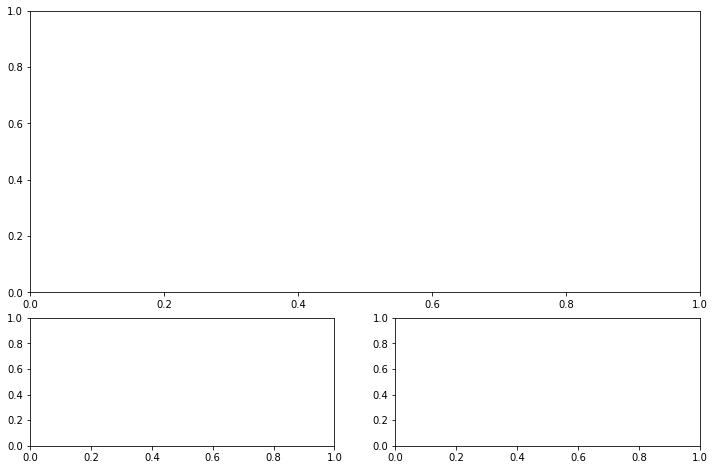In :
ax1.set_title("Home value as a function of home age & area population", fontsize=14)
sctr = ax1.scatter(x=age, y=pop, c=y, cmap="RdYlGn")
plt.colorbar(sctr, ax=ax1, format="\$%d")
ax1.set_yscale("log")
ax2.hist(age, bins="auto")
ax3.hist(pop, bins="auto", log=True)
add_titlebox(ax3, "Histogram: area population (log scl.)")

Out:
<matplotlib.axes._subplots.AxesSubplot at 0x7ff3b8a668c0>In :
fig

Out: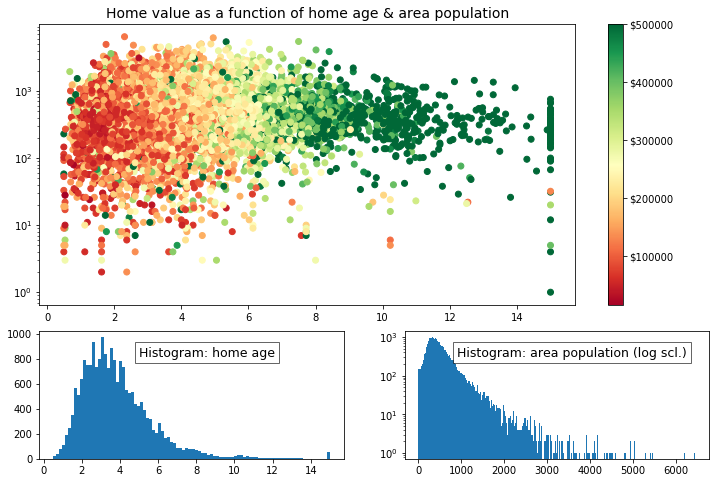In :
[plt.figure(i) for i in plt.get_fignums()]

Out:
[]
In :
plt.get_fignums()

Out:
[]

While ax.plot() is one of the most common plotting methods on an Axes, there are a whole host of others, as well. (We used ax.stackplot() above. You can find the complete list here.)

Methods that get heavy use are imshow() and matshow(), with the latter being a wrapper around the former. These are useful anytime that a raw numerical array can be visualized as a colored grid.

In :
(x := np.diag(np.arange(2, 12))[::-1])

Out:
array([[ 0,  0,  0,  0,  0,  0,  0,  0,  0, 11],
[ 0,  0,  0,  0,  0,  0,  0,  0, 10,  0],
[ 0,  0,  0,  0,  0,  0,  0,  9,  0,  0],
[ 0,  0,  0,  0,  0,  0,  8,  0,  0,  0],
[ 0,  0,  0,  0,  0,  7,  0,  0,  0,  0],
[ 0,  0,  0,  0,  6,  0,  0,  0,  0,  0],
[ 0,  0,  0,  5,  0,  0,  0,  0,  0,  0],
[ 0,  0,  4,  0,  0,  0,  0,  0,  0,  0],
[ 0,  3,  0,  0,  0,  0,  0,  0,  0,  0],
[ 2,  0,  0,  0,  0,  0,  0,  0,  0,  0]])
In :
x[np.diag_indices_from(x[::-1])] = np.arange(2, 12)
x

Out:
array([[ 2,  0,  0,  0,  0,  0,  0,  0,  0, 11],
[ 0,  3,  0,  0,  0,  0,  0,  0, 10,  0],
[ 0,  0,  4,  0,  0,  0,  0,  9,  0,  0],
[ 0,  0,  0,  5,  0,  0,  8,  0,  0,  0],
[ 0,  0,  0,  0,  6,  7,  0,  0,  0,  0],
[ 0,  0,  0,  0,  6,  7,  0,  0,  0,  0],
[ 0,  0,  0,  5,  0,  0,  8,  0,  0,  0],
[ 0,  0,  4,  0,  0,  0,  0,  9,  0,  0],
[ 0,  3,  0,  0,  0,  0,  0,  0, 10,  0],
[ 2,  0,  0,  0,  0,  0,  0,  0,  0, 11]])
In :
(x2 := np.arange(x.size).reshape(x.shape))

Out:
array([[ 0,  1,  2,  3,  4,  5,  6,  7,  8,  9],
[10, 11, 12, 13, 14, 15, 16, 17, 18, 19],
[20, 21, 22, 23, 24, 25, 26, 27, 28, 29],
[30, 31, 32, 33, 34, 35, 36, 37, 38, 39],
[40, 41, 42, 43, 44, 45, 46, 47, 48, 49],
[50, 51, 52, 53, 54, 55, 56, 57, 58, 59],
[60, 61, 62, 63, 64, 65, 66, 67, 68, 69],
[70, 71, 72, 73, 74, 75, 76, 77, 78, 79],
[80, 81, 82, 83, 84, 85, 86, 87, 88, 89],
[90, 91, 92, 93, 94, 95, 96, 97, 98, 99]])
In :
sides = (
"left",
"right",
"top",
"bottom",
)

In :
nolabels = {s: False for s in sides}

In :
nolabels.update({f"label{s}": False for s in sides})

In :
nolabels

Out:
{'left': False,
'right': False,
'top': False,
'bottom': False,
'labelleft': False,
'labelright': False,
'labeltop': False,
'labelbottom': False}
In :
from mpl_toolkits.axes_grid1.axes_divider import make_axes_locatable

In :
with plt.rc_context(rc={"axes.grid": False}):
fig, (ax1, ax2) = plt.subplots(1, 2, figsize=(8, 4))
ax1.matshow(x)
img2 = ax2.matshow(x2, cmap="RdYlGn_r")
for ax in (ax1, ax2):
ax.tick_params(axis="both", which="both", **nolabels)
for i, j in zip(*x.nonzero()):
ax1.text(j, i, x[i, j], color="white", ha="center", va="center")
divider = make_axes_locatable(ax2)
plt.colorbar(img2, cax=cax, ax=[ax1, ax2])
fig.suptitle("Heatmaps with Axes.matshow", fontsize=16)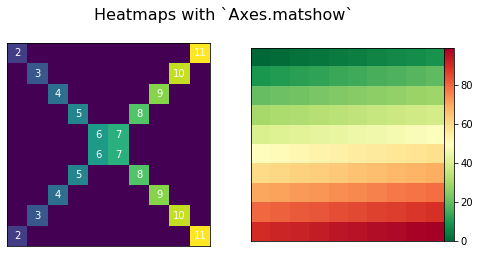The pandas library has become popular for not just for enabling powerful data analysis, but also for its handy pre-canned plotting methods. Interestingly though, pandas plotting methods are really just convenient wrappers around existing matplotlib calls.

In :
import pandas as pd

In :
(s := pd.Series(np.arange(5), index=list("abcde")))

Out:
a    0
b    1
c    2
d    3
e    4
dtype: int64
In :
(ax := s.plot())

Out:
<matplotlib.axes._subplots.AxesSubplot at 0x7ff3adf9ef50>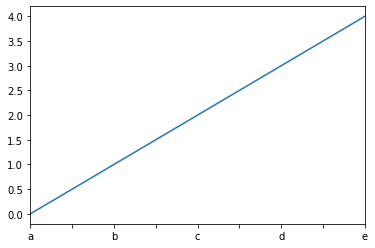In :
type(ax)

Out:
matplotlib.axes._subplots.AxesSubplot
In :
id(plt.gca()), id(ax)

Out:
(140684556626192, 140684572618576)In :
import matplotlib.transforms as mtransforms

In :
url = "https://fred.stlouisfed.org/graph/fredgraph.csv?id=VIXCLS"

In :
vix = pd.read_csv(
url, index_col=0, parse_dates=True, infer_datetime_format=True, squeeze=True
).dropna()

In :
vix = vix[vix != "."]

In :
(ma := vix.rolling("90d").mean())

Out:
DATE
1990-01-02    17.240000
1990-01-03    17.715000
1990-01-04    18.216667
1990-01-05    18.690000
1990-01-08    19.004000
...
2020-02-21    14.044500
2020-02-24    14.310000
2020-02-25    14.582881
2020-02-26    14.799167
2020-02-27    15.241500
Name: VIXCLS, Length: 7595, dtype: float64
In :
{type(item) for item in vix}

Out:
{str}
In :
vix_ = pd.Series([float(item) for item in vix])

In :
state = pd.cut(ma, bins=[-np.inf, 14, 18, 24, np.inf], labels=range(4))
cmap = plt.get_cmap("RdYlGn_r")
ma.plot(color="black", linewidth=1.5, marker="", figsize=(8, 4), label="VIX 90d MA")
ax = plt.gca()  # Get the current Axes that ma.plot() references
ax.set_ylabel("90d moving average: CBOE VIX")
ax.set_title("Volatility Regime State")
ax.grid(False)
ax.legend(loc="upper center")
ax.set_xlim(xmin=ma.index, xmax=ma.index[-1])
trans = mtransforms.blended_transform_factory(ax.transData, ax.transAxes)
for i, color in enumerate(cmap([0.2, 0.4, 0.6, 0.8])):
ax.fill_between(ma.index, 0, 1, where=state == i, facecolor=color, transform=trans)
ax.axhline(
vix_.mean(),
linestyle="dashed",
color="xkcd:dark grey",
alpha=0.6,
label="Full-period mean",
marker="",
)

Out:
<matplotlib.lines.Line2D at 0x7ff3ac632370>